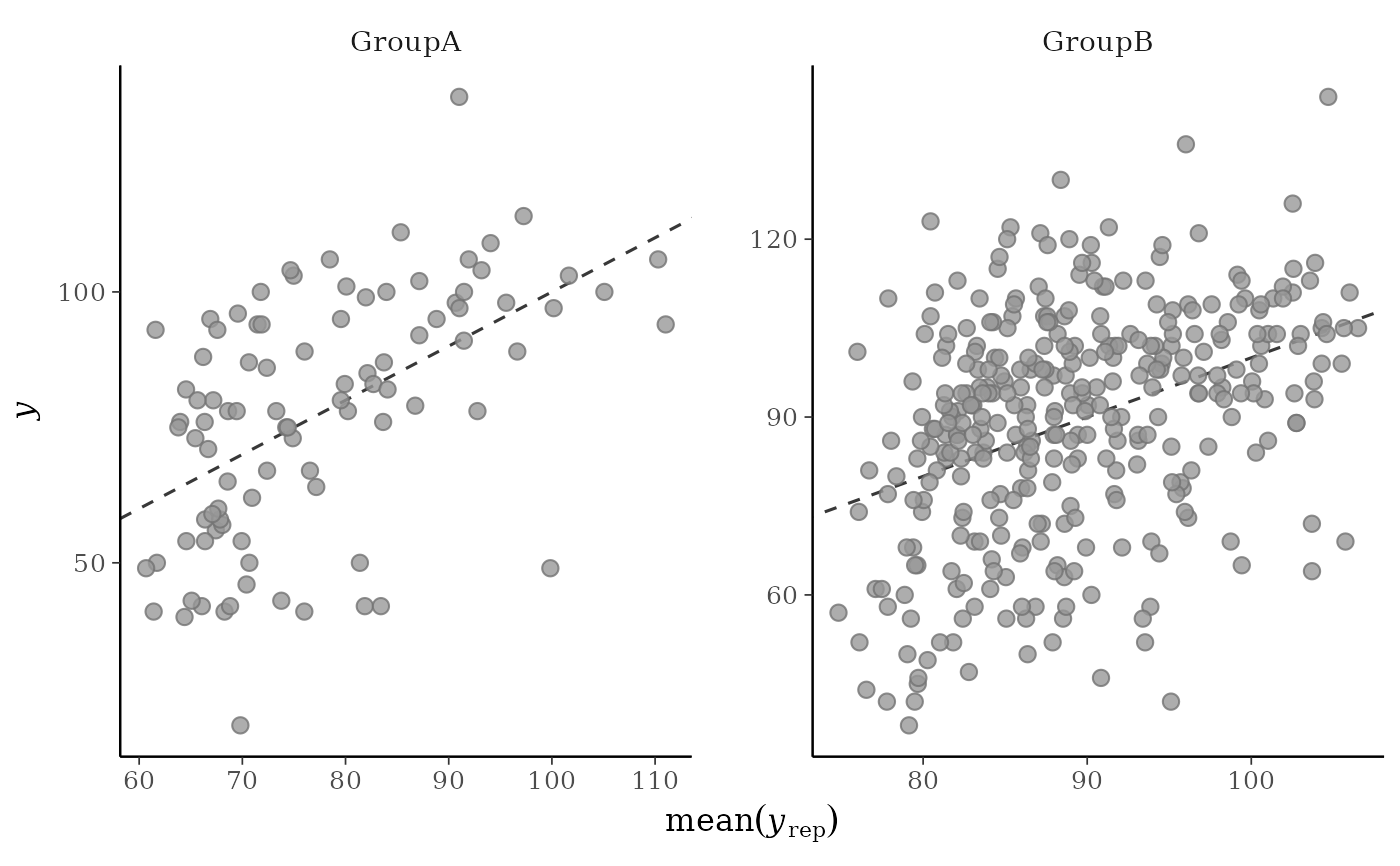Scatterplots of the observed data y vs. simulated/replicated data yrep from the posterior predictive distribution. See the Plot Descriptions and Details sections, below.

ppc_scatter(
y,
yrep,
...,
facet_args = list(),
size = 2.5,
alpha = 0.8,
ref_line = TRUE
)

ppc_scatter_avg(y, yrep, ..., size = 2.5, alpha = 0.8, ref_line = TRUE)

ppc_scatter_avg_grouped(
y,
yrep,
group,
...,
facet_args = list(),
size = 2.5,
alpha = 0.8,
ref_line = TRUE
)

ppc_scatter_data(y, yrep)

ppc_scatter_avg_data(y, yrep, group = NULL)

## Arguments

y

A vector of observations. See Details.

yrep

An S by N matrix of draws from the posterior (or prior) predictive distribution. The number of rows, S, is the size of the posterior (or prior) sample used to generate yrep. The number of columns, N is the number of predicted observations (length(y)). The columns of yrep should be in the same order as the data points in y for the plots to make sense. See the Details and Plot Descriptions sections for additional advice specific to particular plots.

...

Currently unused.

facet_args

A named list of arguments (other than facets) passed to ggplot2::facet_wrap() or ggplot2::facet_grid() to control faceting. Note: if scales is not included in facet_args then bayesplot may use scales="free" as the default (depending on the plot) instead of the ggplot2 default of scales="fixed".

size, alpha

Arguments passed to ggplot2::geom_point() to control the appearance of the points.

ref_line

If TRUE (the default) a dashed line with intercept 0 and slope 1 is drawn behind the scatter plot.

group

A grouping variable of the same length as y. Will be coerced to factor if not already a factor. Each value in group is interpreted as the group level pertaining to the corresponding observation.

## Value

The plotting functions return a ggplot object that can be further customized using the ggplot2 package. The functions with suffix _data() return the data that would have been drawn by the plotting function.

## Details

For Binomial data, the plots may be more useful if the input contains the "success" proportions (not discrete "success" or "failure" counts).

## Plot Descriptions

ppc_scatter()

For each dataset (row) in yrep a scatterplot is generated showing y against that row of yrep. For this plot yrep should only contain a small number of rows.

ppc_scatter_avg()

A single scatterplot of y against the average values of yrep, i.e., the points (x,y) = (mean(yrep[, n]), y[n]), where each yrep[, n] is a vector of length equal to the number of posterior draws. Unlike for ppc_scatter(), for ppc_scatter_avg() yrep should contain many draws (rows).

ppc_scatter_avg_grouped()

The same as ppc_scatter_avg(), but a separate plot is generated for each level of a grouping variable.

Other PPCs: PPC-censoring, PPC-discrete, PPC-distributions, PPC-errors, PPC-intervals, PPC-loo, PPC-overview, PPC-test-statistics

## Examples

y <- example_y_data()
yrep <- example_yrep_draws()
p1 <- ppc_scatter_avg(y, yrep)
p1# don't draw line x=y
ppc_scatter_avg(y, yrep, ref_line = FALSE)p2 <- ppc_scatter(y, yrep[20:23, ], alpha = 0.5, size = 1.5)
p2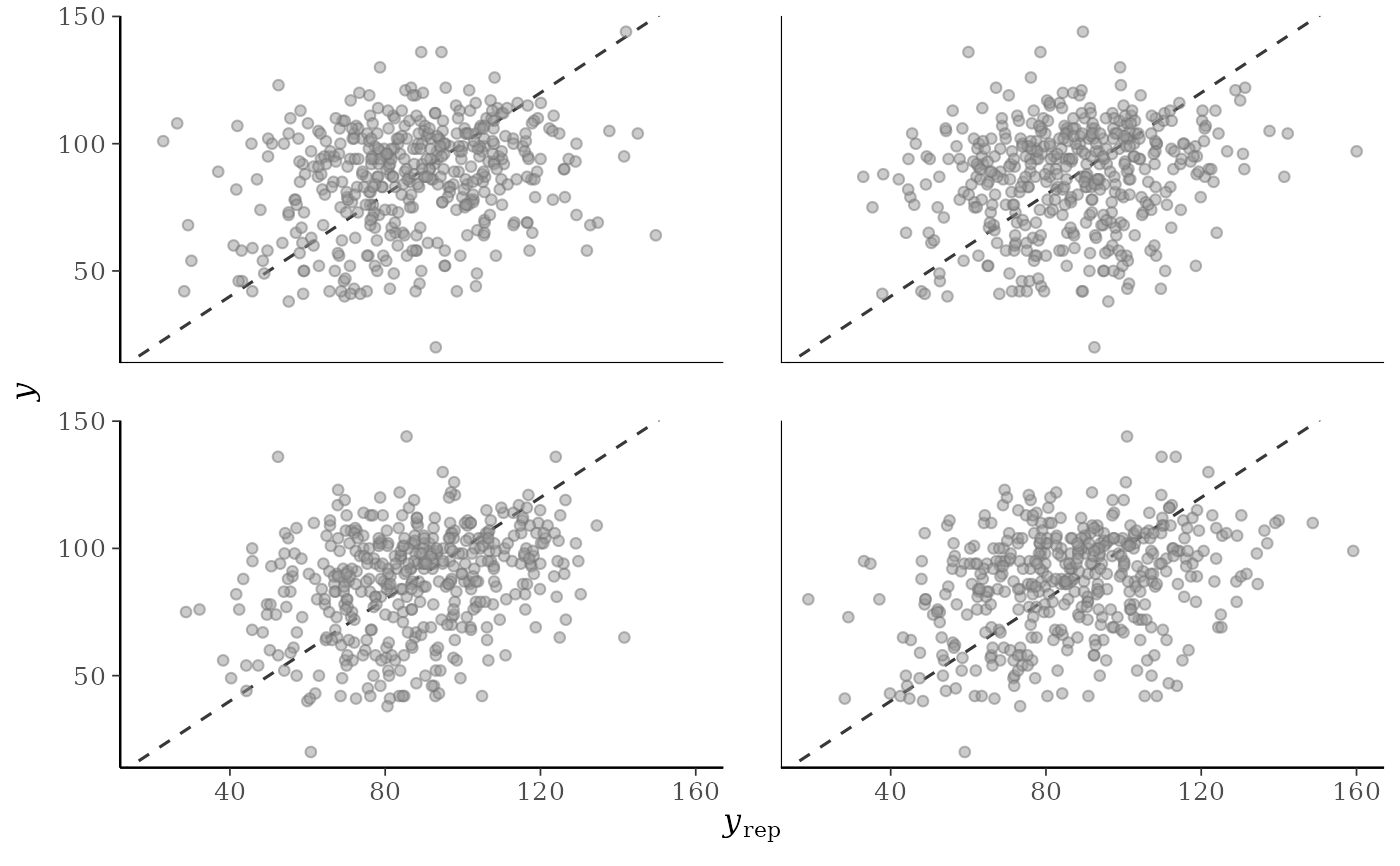# give x and y axes the same limits
lims <- ggplot2::lims(x = c(0, 160), y = c(0, 160))
p1 + lims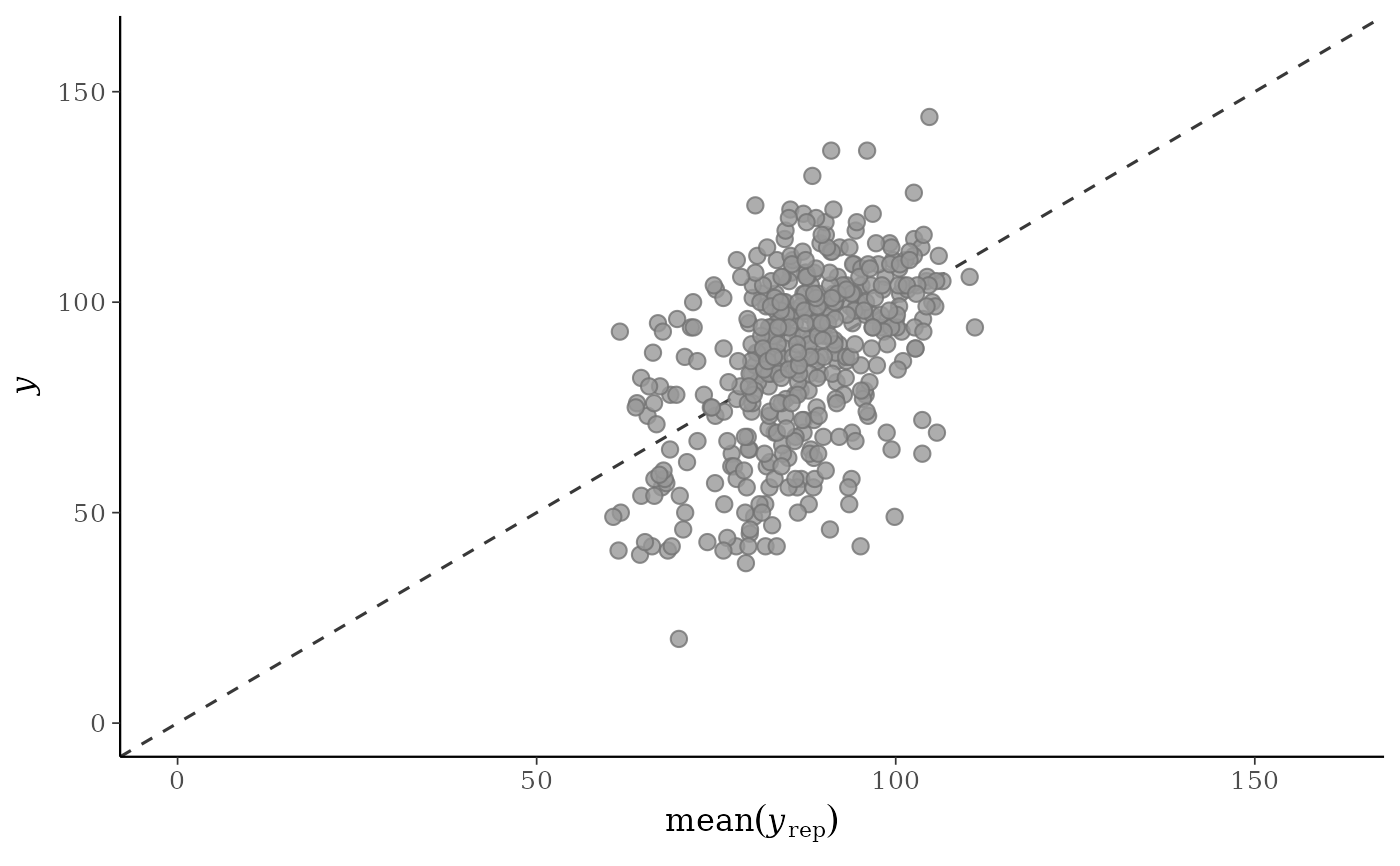p2 + lims
#> Warning: Removed 1 rows containing missing values (geom_point()).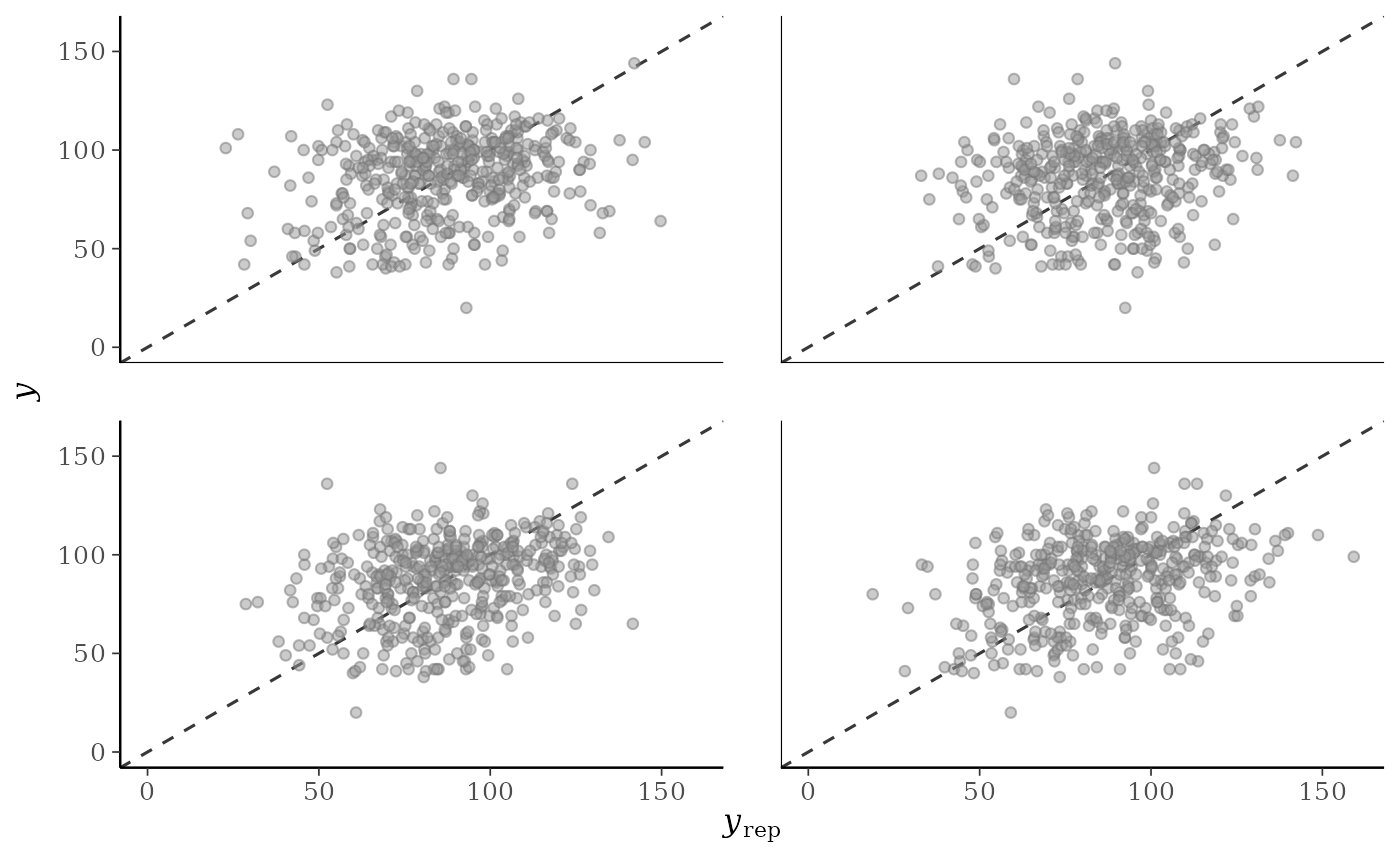# for ppc_scatter_avg_grouped the default is to allow the facets
# to have different x and y axes
group <- example_group_data()
ppc_scatter_avg_grouped(y, yrep, group)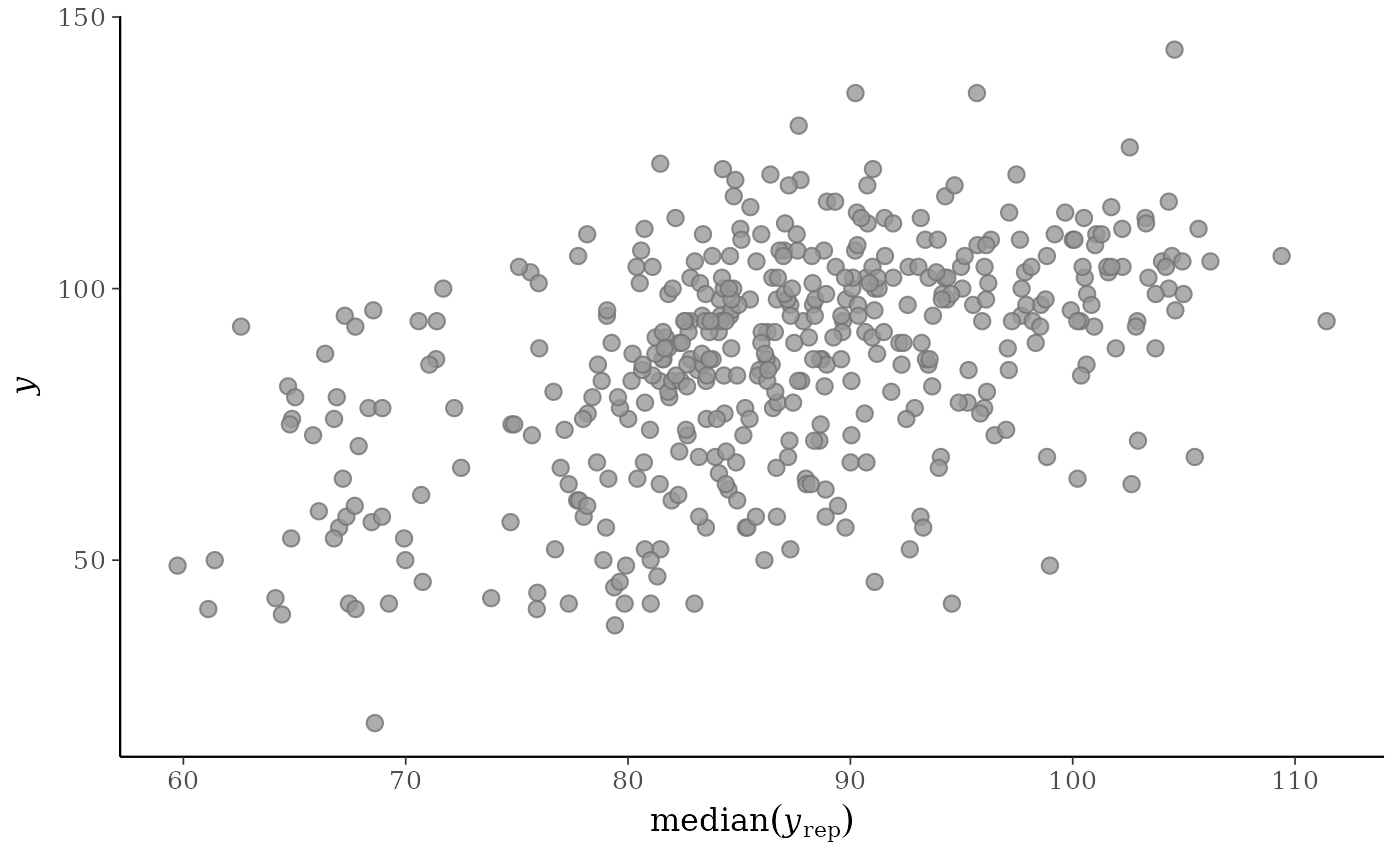# let x-axis vary but force y-axis to be the same
ppc_scatter_avg_grouped(y, yrep, group, facet_args = list(scales = "free_x"))